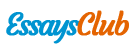# Electrochemistry Analytical Chemistry

Autor:   •  January 15, 2019  •  858 Words (4 Pages)  •  147 Views

Page 1 of 4

...

Galvanic Cells:

For part 1, The theoretical ranking of relative potential: Cu2+ >Fe2+>Zn2+>Al3+>Mg2+. And The actual ranking of relative potential: Cu2+ >Fe2+>Zn2+>Al3+>Mg2+. The ranking of the measure relative potential was the same as the theoretical ranking of relative potential. The measured voltage were close to the predicted values, however, for testing the voltage difference between Al and Cu, there exists significant difference, which might due to the system of voltmeter. In addition, the oxide layer of these two metals caused small voltage.

For part 2, from the data we collected from the lab, we could figure out that the measured and calculated voltages differ significantly. The differences between predicted and measured were around 0.3 voltage, so the error might due to the system of voltmeter.

The voltage difference existed between a cell with copper half cells of different concentrations, which was 0.0704V. In theory, same electrodes provide the same potentials, which should shows Eocell = 0. However, the concentrations are different, which gives the driving force.

Electroplating:

- Q= It = 0.249A x 600 s= 149.4 Coulomb

- Theoretical moles of copper= Q/Fn=149.4/(96485x2)=7.7421x10-4 mol

- Actual moles of copper= (0.4938-0.4248)/63.5= 0.00108 mol

- %yield=actual yield /theoretical yield x 100%

= 0.00108mol/7.768x10-4 mol x 100%

=139%

- The surface of the metals might not be cleaned enough, so there were some impurity on the metals when we measured the mass. The loss of copper includes the loss of impurity, which made the calculated value of the actual mole of copper larger than the theoretical value .

- Oxidation half-cell: Cu → Cu2+ + 2e-

- Reduction half-cell: Cu2+ + 2e-→ Cu

...